# IF Formula with Four Conditions

Many insights derived from data analyses include determining the number of observations that meet specific characteristics. For example, an analysis of a person’s ideal automobile purchase may require that the price is below a specific dollar amount, the engine has a minimum horsepower rating, and an automatic transmission. While it is possible to scan through the data and mark each observation that meets these requirements, a simpler and faster way is to use an IF/AND or IF/OR function combination. This lesson will explain how to utilize this combination properly.

The IF Formula:

=IF(logical_test, [value_if_true], [value_if_false])

Let’s begin with a review of the IF formula by itself. This nifty formula in Excel allows the user to specify a logical condition, either numerical or textual, and returns a value, also specified by the user, for whether the condition is met (TRUE) or not met (FALSE). The statement has three parts, each separated by a comma, as shown in the formula above: the logical test, the value if true, and the value if false. Continuing with the automobile purchase example, take a look at the following worksheet:Please note that the values shown are not actual market data (I made them up!). Regardless, cell H2 shows the IF formula to determine whether or not I can afford the Jeep Patriot. The first part of the formula, the “logical test,” checks to see if the price listed for the Patriot in D2 is less than the amount that I feel comfortable paying: \$20,000. In the next part, I have specified that I want to see the word “YES” if the price is indeed below \$20,000 and the word “NO” if it is not (if it is equal to or greater than \$20,000).

Notice that I used quotation marks around these words. If you wish to have text returned from the IF formula, you must put quotes around the text that you want for the true and false values. A common use of the IF formula in data analytics is for returning binary values of either 1 or 0. For these numerical outputs, quotes are not needed; in our example, simply replace the “YES” with a 1 and the “NO” with a 0 (without quotation marks).

Let’s return to our worksheet and see what happens after we type the formula and hit enter:Because the price of the Patriot in D2, \$25,000, was not less than \$20,000, the formula returned the value that I specified if the condition was not met: “NO.” Now that our formula is set up, we can drag down the column and determine the affordability of each of the listed vehicles:We can see that based on my budget of \$20,000, I can only afford the Honda Civic, Toyota Camry, or Kia Rio. I’m that much closer to finding my ideal automobile, but to truly determine which one I want to buy, I will need to assess more conditions. In the next section of this lesson, we will do this together, where we will combine the IF and AND formulas.

The IF Formula with Multiple Conditions using the AND Formula:

=IF(AND(logical_test 1, logical_test 2, …), [value_if_true], [value_if_false])

As the title of this tutorial suggests, we want to learn how to use the IF formula with at least four conditions. So far, we have seen one condition: the price must be less than \$20,000. However, like many other data analyses, my ultimate decision is not determined by one aspect of the observations alone. Beyond making a purchase that fits my budget, I also would like a vehicle with the model year of 2013 or newer, a horsepower of at least 145, and an automatic transmission. These three characteristics will be the additional conditions the vehicles will need to fulfill to be ideal for purchase.

Notice that all of these conditions must be met for the vehicle to be ideal for purchase. There are multiple ways to determine such things in Excel, including (the utterly barbaric way of) scanning through the data and manually marking those that meet all of the conditions or creating an IF formula in each column that focuses on a single condition and then aggregating those results. Neither of these methods is as efficient and elegant as combining the AND formula with the IF formula.

Look at the worksheet below. You’ll see that I have added another column, “Ideal,” next to that of “Can I Afford?” where I determined whether each vehicle was in my price range:The formula in cell I2 may look a bit hairier than the one we saw before in H2, but I have to tell you, with utmost sincerity, that it is not. Below I have written the formula for the IF/AND combination from I2:

=IF(AND(D2<20000,C2>2012,E2>145,F2=“Automatic”), “YES”, “NO”)

Now watch this formula transform into our original simple IF formula that checked each vehicle’s price condition:

=IF(AND(D2<20000,C2>2012,E2>145,F2=“Automatic”), “YES”, “NO”)

Here’s the secret: the AND formula takes the place of the logical test in the IF formula. Then, within the AND formula's parenthesis, you can write as many conditions (logical tests) as you want, each separated by a comma. The AND formula by itself will return either a “TRUE” if all the logical tests are met or a “FALSE” if even one is not met. As such, the IF formula around the AND will let you specify your values for a return of “TRUE” or “FALSE,” as we did in the first section.

Okay, now let’s see what happens when I hit enter, and we assess whether or not the Jeep Patriot is the ideal vehicle for me:“NO.” This outcome should not surprise anyone. Recall that my ideal vehicle has four conditions:

1) It costs less than \$20,000:                            D2<20000

2) It is newer than a 2012 model:                   C2>2012

3) It has at least 145 horsepower:                 E2>145

4) It has automatic transmission:                   F2=“Automatic”

While it does pretty well, hitting points 2 through 4, it fails the first condition, that of price. It is not ideal as I have defined it. Now let’s drag down the formula for each of the other nine vehicles and see if there are any that I would consider ideal:PHEW! Thank Goodness! Row 9 contains a Kia Rio that returned a “YES.” Let’s see why:

1) It costs less than \$20,000:                             D9<20000

2) It is newer than a 2012 model:                     C9>2012

3) It has at least 145 horsepower:                   E9>145

4) It has automatic transmission:                     F9=“Automatic”

Looks like I’ve got a sporty Rio in my future.

The IF Formula with Multiple Conditions using the OR Formula:

=IF(OR(logical_test 1, logical_test 2, …), [value_if_true], [value_if_false])

Now that we’ve reviewed IF formulas and the IF/AND combination, we can quickly cover another combination: the IF/OR formula. Take a look at the equation above. Look familiar? It should. In structure, this combination is exactly the same as its AND-containing cousin. The difference is in its operation. Whereas the IF/AND combination will only return the value that you specify for “TRUE” if ALL of the logical arguments hold true, the IF/OR combination will return the value that you specify for “TRUE” if ANY of the logical arguments are true.

Going back to my vehicle purchase example, I’ve just realized that my conditions have led me to an ideal vehicle that I never thought I’d own in a million years: a Kia Rio. Therefore, I decided that I needed to reevaluate my criteria. I determine that horsepower and model year are immaterial. Again I focus on my budget and am rather adamant about not going above \$20,000. However, I would be willing to break this budget if I could get the vehicle with an automatic transmission. Here’s the spreadsheet again:Notice that “AND” has been replaced by “OR,” and I have dropped two of the four logical tests so that only price and transmission are being assessed, but otherwise, the formula follows the same structure. Let’s skip ahead and apply the formula to each vehicle:We can see a few cells containing “YES” in this column, including the Jeep Patriot. Let’s see why:

1) It costs less than \$20,000:                     D9<20000

2) It has automatic transmission:             F9=“Automatic”

While the Jeep had been excluded on price in the first and second analyses, our swap of “AND” for “OR” allowed the program to return a “TRUE” value (a “YES”) because the Jeep did meet one of the conditions, an automatic transmission.

## Use Multiple IF Conditions in Nested Form in Excel

Assuming you have grades of the different students according to their total marks.

1. Go ahead and highlight cell F6 and type the formula;

=IF(E4>160,"A",IF(E4>=150,"B",IF(E4>140,"C",IF(E4>130,"D",IF(E4<130,"F")))))2. Tap Enter button, which places the values of Grades A in cell F6.

3. Drop the Fill Handle tool downwards from cell F4 to F13.From the example, you will note that;

• The formula brings back grade A values when the totals are more than or equal to
• It brings back a grade of B if the total mark is more than or equal to
• Returns grade C when the total mark is more than or equal to
• It brings back a grade of D if the total mark is more than or the same as
• Returns grade F when the total mark is less than

## IF Functions With Multiple Outcomes

### Inserting AND & OR Logic with Multiple IF Conditions

1. Start by highlighting cell E4.

2. Compute the formula below in the cell;

=IF(OR(AND(C4>=90, D4>=80), AND(C4>=60, D4>=50)), "Pass", "Fail")3. Press the Enter button.

5. Drop the Fill Handle tool from E4 to E13.6. Finally, a similar image emerges.

The formula will bring the results Pass when the values in the cells C4 & D4 respectively is >=90 & >=80.Both the results >=90 & >=80 and >=60 & >=50 are same.

When neither of the other values fits the condition, you will regard them as failures.

### Applying Multiple IF Conditions with Different Excel Functions

In this method, you will use the SUM function.

Steps;

1. Begin by highlighting cell C14.

2. Continue by placing the formula below:

=IF(SUM(C4:C13)>=350, "Good", IF(SUM(C4:C13)>=300, "Satisfactory", "Poor "))

3. Tap the Enter button.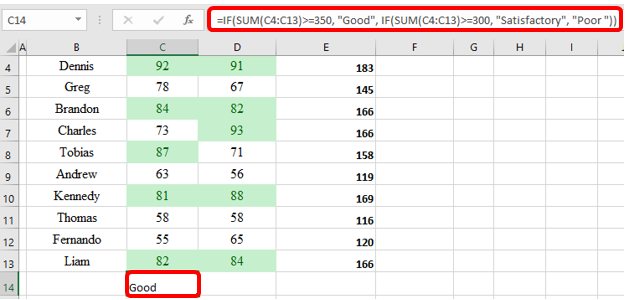4. You will score Good in cell C14.

5. Right-click the Fill Handle tool horizontally from cell C14 to E14.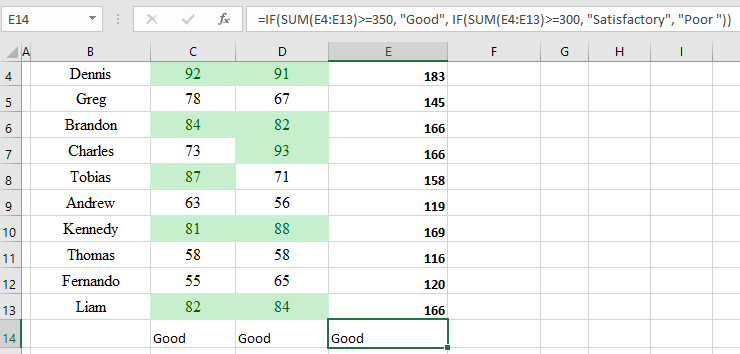According to your formula, you should expect the bring-back value to be Good. However, SUM(C4:C13) is more than or the same as 350.

It takes back Satisfactory if the value of SUM(C4:C13) is more than or the same as 300.

In case the value of SUM(C4:C13) is less than 300, the answer will be Poor.

### IF with SUM, AVERAGE, MIN, and MAX Functions

Generally, in this method, SUMIF and SUMIFS functions are used.

Steps;

1. In your business, sometimes you need to use the SUM function to try and identify IF. Since the return of many texts relies on the sum of values in B2 and C2.

2. You will use the formula below;

=IF(SUM(C4:D4)>130, "Good", IF(SUM(C4:D4)>110, "Satisfactory", "Poor"))

When the results show totals of more than 130, then the answer is “good.” Since the result is more than 110, it’s satisfactory, but less than 110, the result will be “poor.”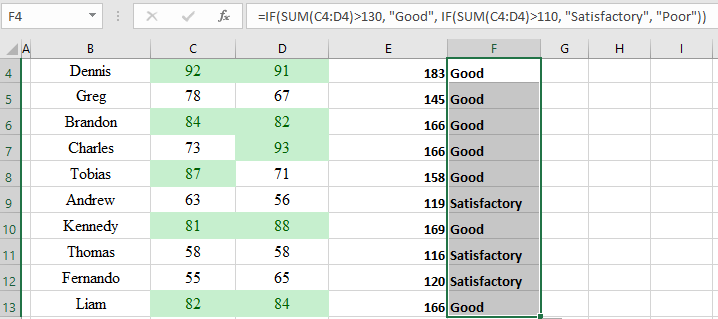On the contrary, you can also use the Average function where;

=IF(AVERAGE(C4:D4)>65, "Good", IF(AVERAGE(C4:D4)>55, "Satisfactory", "Poor"))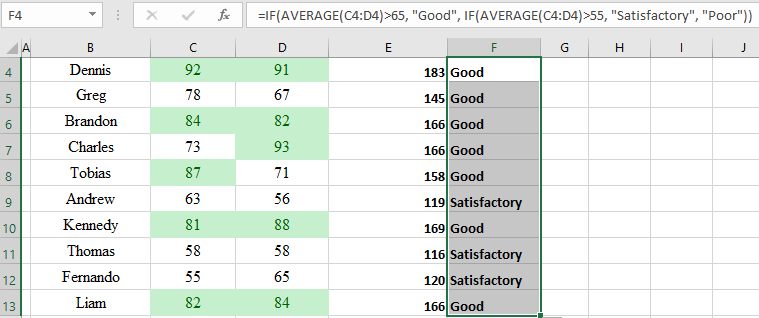Assume that in Column F you want to select the highest and lowest values with MAX and MIN functions;

=IF(D4=MAX(\$D\$4:\$D\$13), “Best result,” “”)

=IF(D4=MIN(\$D\$4:\$D\$13, ”Worst result,” “”)

As well, you can state them in one column as a combined formula;

=IF(D4=MAX(\$D\$4:\$D\$13), "Best result", IF(D4=MIN(\$D\$4:\$D\$13), "Worst result", ""))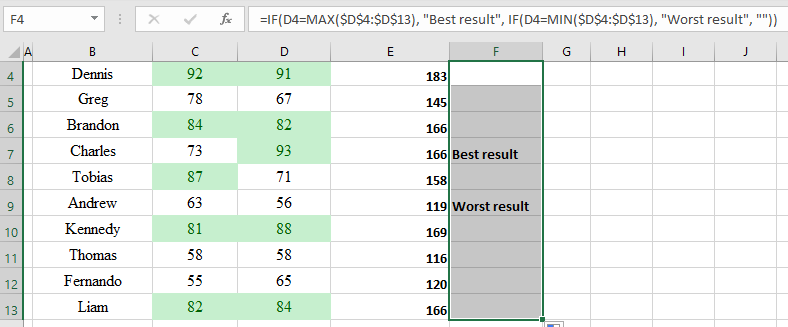IF can also be combined with GetCellColor or GetCellFontColor to identify other results depending on cell color.

### A Quick Summary:

Note the differences between the cells in the “Can I Afford?,” “Ideal,” and “Good Enough” columns in the last graphic.

In the first, we used the IF formula to determine if we could afford each automobile; if the price was less than \$20,000. With this simple, single-condition IF formula, we found that the Honda, Toyota, and Kia would be affordable purchases.

In the second, we sought our ideal vehicle by specifying that we wanted to see a “YES” if it were less than \$20,000, newer than 2012, had at least 145 horsepower, and had an automatic transmission. We found that one car was ideal: the Kia Rio.

In the third, we decided that horsepower and transmission were unimportant. Instead, we decided that we did not want to spend more than \$20,000 unless the vehicle had an automatic transmission. This allowed for several other options besides those that we determined we could afford under the first column; the Jeep, Subaru, and Lincoln are all considered good enough because they have automatic transmissions (though they are still overpriced).